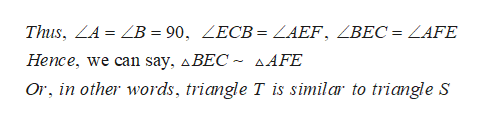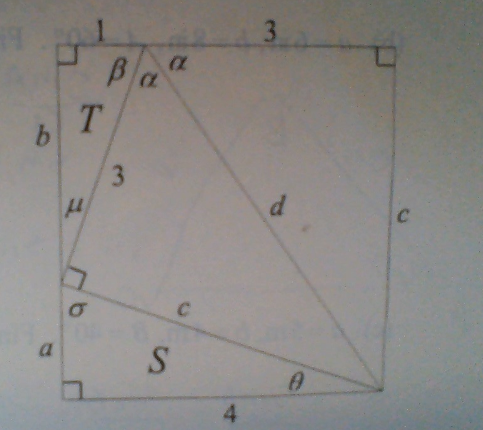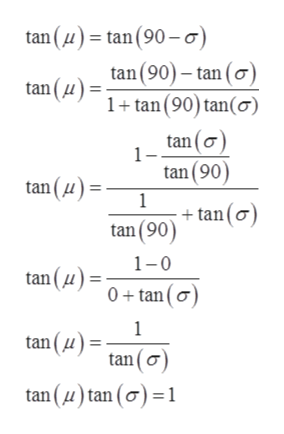# Thus, ZA = ZB = 90, ZECB = ZAEF, ZBEC = ZAFEHence, we can say, ABEC ~ sAFEOr, in other words, triangle T is similar to triangle S ВаTbCсS4Пь

Question
53 views

A rectangular strip of paper of width 4 inches is folded on the dashed line. See the diagram. Certain angles are angled to help solve solutions in part a (show that triangle T is similar to triangle S, answer attached in photos) and part C:

part C: use the diagram to show that tan^-1 2sqrt2 + 2tan^-1 sqrt2 = pi (radians).

Use only the diagram, basic angle facts, and the definitions of tan and tan^-1. In particular, do not use a calculator.help_outlineImage TranscriptioncloseThus, ZA = ZB = 90, ZECB = ZAEF, ZBEC = ZAFE Hence, we can say, ABEC ~ sAFE Or, in other words, triangle T is similar to triangle S fullscreenhelp_outlineImage TranscriptioncloseВа T b C с S 4 Пь fullscreen
check_circle

Step 1

A rectangular strip of paper of width 4 inches is folded on the dashed line.

Step 2

From the geometry, the angles µ and σ are in linear, so

Step 3

Taking tan of bo...help_outlineImage Transcriptionclosetan (u)tan (90-o tan(90)- tan (o) 1tan (90) tan(o) tan (u) tan(o tan (90) 1 +tan(o) tan (90) tan (u) 1-0 tan (4) 0+tan(o 1 tan (u) tan (o tan (u)tan (o)1 fullscreen

### Want to see the full answer?

See Solution

#### Want to see this answer and more?

Solutions are written by subject experts who are available 24/7. Questions are typically answered within 1 hour.*

See Solution
*Response times may vary by subject and question.
Tagged in

### Trigonometry